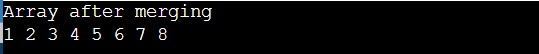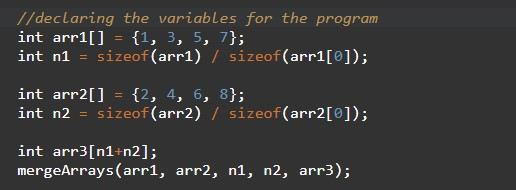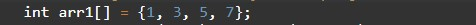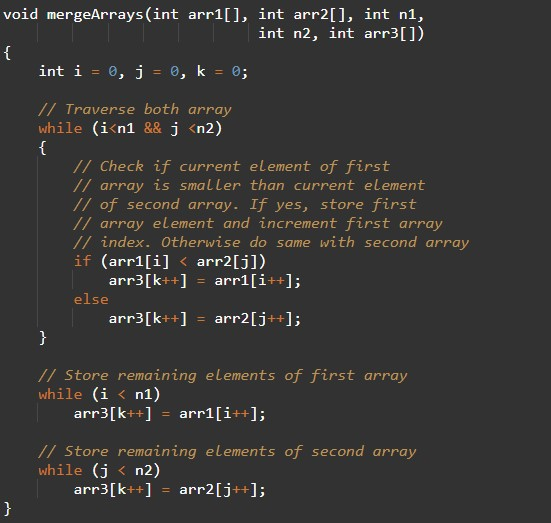# c++ program to merge two arrays

In this tutorial you will learn about the c++ program to merge two arrays and its application with practical example.

## C++ Program to Merge Two Arrays

In this tutorial, we will learn to create a C++ program that will merge two arrays using C++ programming.

## Prerequisites

Before starting with this tutorial we assume that you are best aware of the following C++ programming topics:

• Operators in C++ Programming.
• Basic Input and Output function in C++ Programming.
• Basic C++ programming.
• For loop in C++ Programming.

## Merging of two arrays:-

In Today’s we will merge two different arrays into a single array with a very simple and shortcode. First, we will take two arrays in input and then we will merge them into one single array using short C++ programming.

## Program:-

To find Merge the two array elements into a single array.

## Output:-In the above program, we have first initialized the required variable• arr1[] = it will hold the elements in an array1.
• arr2[] = it will hold the elements in an array2.
• arr3[] = it will hold the elements in an array3.
• mergeArrays[] = it will hold the elements in an array.
• i = it will hold the integer value to control array using for loop.
• j = it will hold the integer value to control array using for loop.
• k = it will hold the integer value to control array using for loop.

Taking input for array1 as well as array2.Merging the two arrays.Main function Code.In this tutorial we have learn about the c++ program to merge two arrays and its application with practical example. I hope you will like this tutorial.